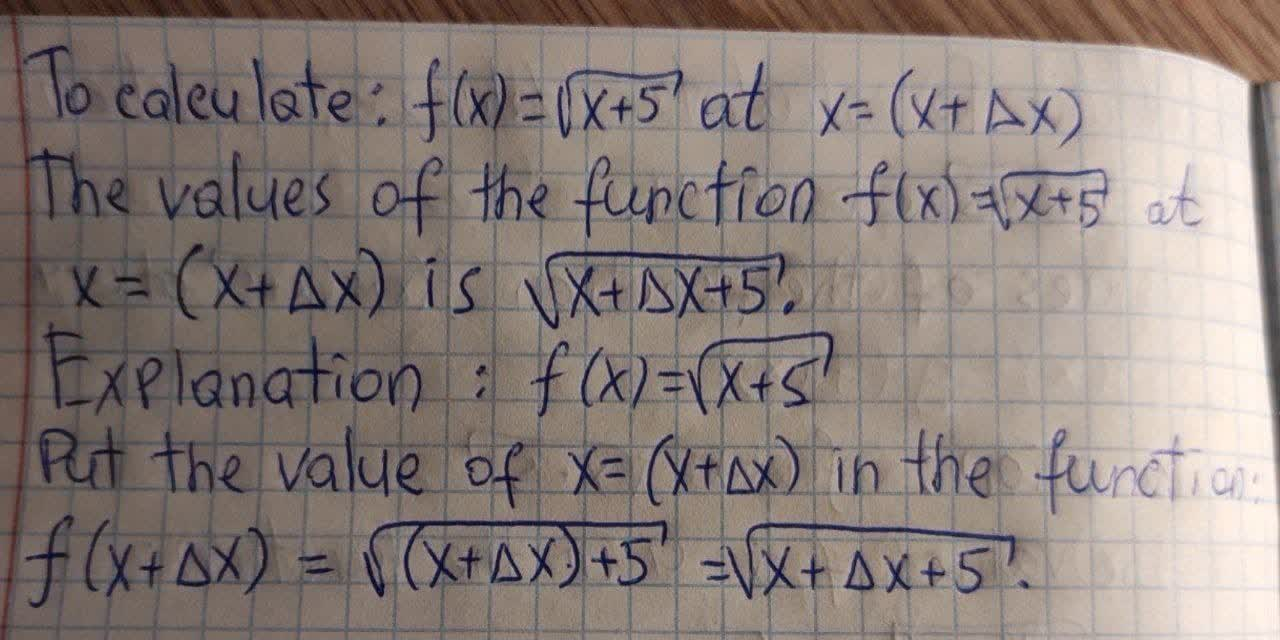# Evaluate the function at given value of the independent variable. Simplify the results.f(x)=sqrt(x+5) f(x+triangle x)Globokim8 2021-09-17 Answered
Evaluate the function at given value of the independent variable. Simplify the results.
$$\displaystyle{f{{\left({x}\right)}}}=\sqrt{{{x}+{5}}}$$
$$\displaystyle{f{{\left({x}+\triangle{x}\right)}}}$$

• Questions are typically answered in as fast as 30 minutes

### Plainmath recommends

• Get a detailed answer even on the hardest topics.
• Ask an expert for a step-by-step guidance to learn to do it yourself.bahaistag

Explanation is on photo